American Journal of Theoretical and Applied Statistics
Volume 4, Issue 3, May 2015, Pages: 185-191

Modeling Panel Data: Comparison of GLS Estimation and Robust Covariance Matrix Estimation

Victor Muthama Musau, Anthony Gichuhi Waititu, Anthony Kibira Wanjoya(V. M. Musau)

Victor Muthama Musau, Anthony Gichuhi Waititu, Anthony Kibira Wanjoya. Modeling Panel Data: Comparison of GLS Estimation and Robust Covariance Matrix Estimation. American Journal of Theoretical and Applied Statistics. Vol. 4, No. 3, 2015, pp. 185-191. doi: 10.11648/j.ajtas.20150403.25

Keywords: Panel, Heteroscedasticity, Autocorrelation, Homogeneity

1. Introduction

Panel data refers to a data containing both time series dimension and cross section dimension in such a way that there are N entities followed over T time periods. If the time periods for which we have data are the same for all N individuals then we have a balanced panel. For cases where the time periods or the length of the time series varies, the data set is referred to as an unbalanced panel. Panel data analysis is at the breaking point of time series and cross-section data analysis.

By combining the time series and cross-sectional dimensions, panel datasets have enabled researchers to study dynamic relationships and to model the heterogeneity among subjects. Most of research works have found their original motivation in panel studies due to their greater capacity for modeling the complexity of human behavior than a single cross-section or time series data. Other areas of studies have showed their interests in analyzing panel data as a way to remove components of variance and estimate causal models.

2. Review of Previous Studies

The term panel study firstly came about in a marketing context when Lazarsfeld and Fiske (1938) considered the effect of radio advertising on product sales. Usually, hearing radio advertisements was thought to increase the possibility of purchasing a product. Lazarsfeld and Fiske sought to find out whether those who bought the product would be more likely to hear the advertisement, or vice versa. They proposed repeatedly interviewing a set of people (the ‘panel’) to clarify the issue. For the first time Marschak (1939) suggests a method to combine cross section and time series information in demand studies. In his later studies Marschak states that "pooling" is the answer to the discussion as to whether cross section or time series methods of demand analysis are preferable Marschak (1943).

The findings of Tobin (1950) contend that the demand function should be consistent with both kinds of observations. He points out that there are both economic and statistical reasons for basing quantitative demand analysis on a combination of time series and cross section data. Wishart (1938), Rao (1965), Potthoff and Roy (1964) introduced the use of multivariate analysis for analyzing growth curves. Particularly, they considered the problem of fitting polynomial growth curves of serial measurements from a group of subjects. Grizzle and Allen (1969), made advancements on this approach by introducing covariates, explanatory variables, into the analysis. Laird and Ware (1982) made the other significant transition from multivariate analysis to regression modeling by introducing the two-stage model that allows for both fixed and random effects.

The need for efficient and consistent estimates challenged the ingenuity of many researchers to come up with models that yielded consistent and efficient estimates. Kleiber and Zeileis (2010) follow the history of Grunfeld data and present replication files to selected results from various studies as well as for the Grunfeld's thesis. In this study GLS and robust covariance matrix estimation techniques were used to model this data set.

3. Methodology

3.1. The Linear Panel Model

The basic linear panel models can be described through suitable restrictions of the following general model: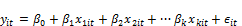(1)

Where i=1, 2…n is the individual (group, state...e.t.c) index, t=1, 2…T is the time index and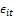is a random disturbance term of mean zero. To model for individual heterogeneity, one often assumes that the disturbance term comprises of two separate components, one of which is specific to the individual and doesn't change over time (time invariant). This is usually referred to as the unobserved heterogeneity component. The other component, conventionally known as the idiosyncratic error component is usually assumed to be independent of both the regressors and the individual error components.

3.2. Generalized Least Squares (GLS)

GLS is normally designed to produce an optimal unbiased estimator of β for the situation with heterogeneous variance. The standard linear model to be considered is of the form: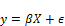(2)

where y is the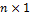response vector, X is a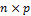model matrix,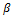is a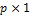vector of parameters to be estimated andis avector of errors.

3.2.1. Parameter Estimation

To obtain the parameter estimates for model … we use the maximum likelihood approach, where we write the log-likelihood of a single observation i (where i=1, 2 ...n) in terms of the unknown regression parameter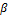and the variance covariance matrix,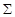;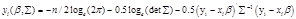(3)

The log-likelihood for the entire dataset becomes;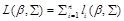(4)

The vector of scores is now calculated by taking the derivatives with respect to the parameters to obtain;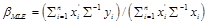(5)

3.2.2. Estimation of  Using FGLS

To generate the estimate for the variance-covariance matrix,, OLS was first applied to the model. This gave consistent estimates of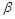and the residuals were then estimated as;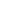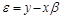(6)These residual values are also expected to be consistent and they are therefore, used to estimate the variance-covariance matrix by multiplying a vector of the residuals with its transpose.

3.3. Robust Covariance Matrix Estimator

Panel data sets are characterized by autocorrelation and heteroscedasticity of unknown form thus resulting to non-spherical disturbances. To make inference from the models generated by such data sets, it is essential to use robust covariance matrix estimators that can consistently estimate the covariance of the model parameters.

Suitable heteroscedasticity consistent (HC) and heteroscedasticity and autocorrelation consistent (HAC) estimators received much attention in the panel literature Zivot and Wang (2003).

3.3.1. Eicker-White Heteroscedasticity Consistent (HC) Covariance Matrix Estimate

Generally, the error-covariance matrix for heteroscedastic and non-autoregressive errors models is given by;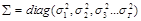(7)

If the nature and form of heteroscedasticity is not known then efficient GLS estimator cannot be computed. However, if the OLS estimator is to be obtained then a consistent estimate for the generalized OLS covariance matrix is needed for proper inference.

A heteroscedasticity consistent estimate of the asymptotic variance matrix of the OLS estimate due to Eicker (1967) and White (1980) is;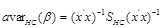(8)

where;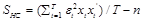(9)

Taking the square root of the diagonal elements of the asymptotic variance matrix estimate gives the Eicker-White heteroscedasticity consistent standard errors (HCSEs) for the least squares estimates.

A number of heteroscedasticity consistent estimators of the asymptotic variance matrix have been proposed whose difference is on the effect imposed to the squared OLS residual. They include;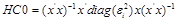(10)

This estimator is arrived at by placing the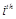squared error into therow of the diagonal of the variance-covariance matrix, using the OLS residuals as estimators of the errors. The entries on the main diagonal of this estimator are the estimated squared standard errors of the regression coefficients.

The second estimator on the other hand, given by;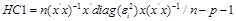(11)

focuses on adjusting the degrees of freedom for HC0. For HC1 every squared OLS residual is now multiplied by a factor of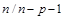.

The third estimator is closely similar to HC1 but instead of a degree-of-freedom adjustment, thesquared OLS residual is now weighted by the reciprocal of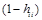where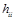are the leverage values and are the diagonal elements of the matrix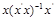.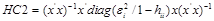(12)

The use of leverage adjusted residuals is justified by the finite-sample bias of HC0 (Cribari-Neto, 2004 and Carroll, 2001) which is touted to be caused by the existence of points of high leverage in thematrix.

The fourth estimator suggest to weight each squared OLS residual by the reciprocal of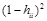rather than.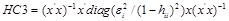(13)

This estimator guarantees best performance to small samples as it gives less weight to high influential observations, Long and Ervin (2000).

The most recent estimator, HC4, derived by Cribari - Neto (2004) was developed with the aim of taking large leverage values into consideration before constructing the asymptotic variance matrix estimator.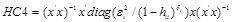(14)

where;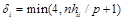The exponent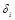controls the "discounting" level for theobservation and with the point of truncation set at 4; the maximum amount of weighting is twice as much as HC3.

3.3.2. Newey-West Heteroscedasticity and Autocorrelation Consistent (HAC) Covariance Matrix Estimate

In several applications of panel regression, it is realized that the disturbance term may be both serially correlated and conditionally heteroscedastic. In such cases the error covariance matrix happens to be non-diagonal.

Considering certain assumptions about the nature of the error heteroscedasticity and serial correlation a consistent estimate of the generalized OLS covariance matrix can be computed. The most popular estimate, due to Newey and West (1987) has the form;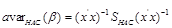(15)

where;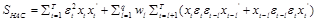Is the estimated, long-run, non-parametric variance and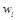is the Bartlett weight function given by;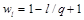4. Results and Discussion

4.1. Introduction

This study used secondary datasets named Grunfeld in R under the package AER. To aid in the analysis of our datasets, several packages including, "nlme", "car", "AER", "zoo", "plm", "systemfit" and "lmtest" were loaded. "Sandwich" package was also employed when estimating the robust covariance matrix estimates. Descriptive analysis was performed and then specification testing of the panel model which involved testing for poolability, for individual or time unobserved effects and for correlation between the individual specific component and the regressors was then carried out before fitting the suggested models.

4.2. Descriptive Statistics

A descriptive analysis was first carried out with the aim of exploring our data sets in order to understand the underlying complex relationships. A coplot was first obtained which is a "conditioning" plot to show the distribution of investments over different years given the firms. Each bar of the eleven conditioning variables (firm) corresponds to one scatter plot. This correlation begins on the left-hand side of figure 1 for the firms, and this first bar (AR) corresponds to the scatter plot on the left-hand side. The scatter plots are then read from left to right.

Heterogeneity plots were also obtained and non-constant average of investments across the firms and the years as shown in figure 2 and figure 3 proved the presence of heterogeneity.Figure 1. Coplot for Grunfeld data set.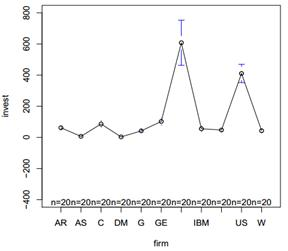Figure 2. Heterogeneity across the Firms.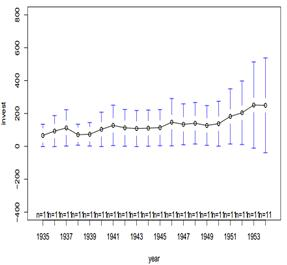Figure 3. Heterogeneity across the Firms.

4.3. Specification Test Results

4.3.1. Test for Poolability

The application of Pooltest on our datasets returned an observed F-statistic of 5.72distributed as F (20,187). The associated p-value is less than 0.0001leading to rejection of the null hypothesis implying that our model parameters; constant and slope coefficients vary across individual firms and therefore individual effects have to be considered.

4.3.2. Tests for Individual and Time Effects

A test for the presence of individual and time effects in our datasets returned a chi-square test statistic of 874.752, with a p-value less than 0.0001 leading to rejection of the null hypothesis in favor of the alternative hypothesis. The study therefore, concludes that there are significant individual and time effects in the residuals.

After establishing the presence of individual and time effects in the residuals, we now check on the relationship between the individual-specific component and the regressors. This is with the aim of finding the optimal model for our datasets between random effects model and fixed effects model. Our choice between fixed and random effects specifications is based on Hausman-type tests, which compares the two estimators under the null of no significant difference (Hausman 1978).

Application of the Hausman test to our datasets returned a chi-square test statistic of 3.9675with a p-value of 0.1376which is greater than 0.05thus we fail to reject the null hypothesis. The study therefore, concludes that endogeneity is not a problem for the random effects estimator implying that both random and fixed effects estimators are consistent but random effects estimator is efficient.

4.3.3. Tests for Cross-Sectional Dependence

Application of this test to our data sets returned a test statistic, Z=5.695and an associated p-value less than 0.0001leading to rejecting the cross-sectional independence hypothesis of the test.

4.3.4. Tests for Heteroscedasticity

Application of the Breusch-Pagan test of homoscedasticity in our data sets returned a test statistic, BP=342.2976and an associated p-value less than 0.0001leading to rejection of the homogenous hypothesis.

The study therefore, concludes that our residuals have non-constant variance across all the cross-sections. This can further be examined by looking at the group specific variances as shown in table 1.

From the results in table 1, sandwich estimators returned the smallest variances followed by the estimators under heteroscedastic feasible generalized least squares and then the estimators under pooled ordinary least squares except for a few firms where this was not the case. Three firms; General motors, US steel and General electric returned the highest variances indicating that they had the biggest difference between the predicted values and the observed values as compared to the other firms.

Table 1. Group Specific Variances under different Models.

 Firms OLS Het FGLS Sandwich General Motors 22289.39 41092.053 22459.814 US Steel 30271.57 40175.892 9130.961 General Electric 34204.25 22528.607 2753.116 Chrysler 452.894 578.942 187.501 Atlantic Refining 3673.75 736.040 162.188 IBM 138.173 71.976 23.276 Union Oil 803.455 97.420 30.739 Westinghouse 2383.15 1549.59 296.051 Goodyear 2529.13 836.079 138.454 Diamond Match 34.533 27.264 3.748 American Steel 147.524 58.039 8.927

The heteroscedasticity and autocorrelation so far established leads to non-spherical disturbance. We therefore, result to using conventional regression-based strategies to address this problem.

4.4. Generalized Least Squares Results

The parameter estimates of the GLS model fitted in our data sets are as shown in table 2.

Table 2. Parameter Estimates of GLS Model.

 Value Std.Error t-value p-value Value 0.09149933 0.00719557 12.716067 0 Capital 0.28637486 0.03385900 8.457866 0

From the results in table 2 we note that both of the regressor variables (value and capital) have p-values which are less than 0.05and therefore they are significant in explaining the dependent variable of our data sets (investment). Both value and capital influence investment positively as their associated coefficients have a positive magnitude. A single unit change in value which is marked as the price of common shares causes investment, defined as additions to plant and equipment plus maintenance and repairs, to increase by 0.09149933millions of dollars. Also a single unit change in capital, marked as the stock of plant and equipment, causes investment to increase by 0.28637486millions of dollars.

The resulting fitted GLS model is;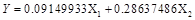where;

Y=investment, X1=Value, X2=Capital

4.5. Feasible Generalized Least Squares Results

The GLS results obtained are based on the assumption of known variance-covariance matrix of the error term, which is usually not the case. In a scenario where this matrix is not known, one has to result into using feasible GLS where ordinary least squares is first applied to the model and then the residuals are estimated. These residuals are expected to be consistent and they are used to estimate the variance-covariance matrix.

The parameter estimates of the FGLS model fitted in our data sets are as shown in table 3.

Table 3. Parameter Estimates of FGLS Model.

 Value Std.Error t-value p-value Value 0.103122 0.003957 26.06 < 2.0×10-16 Capital 0.116644 0.011540 10.11 < 2.0×10-16

From the results in table 3 we note that both of the regressor variables (value and capital) have p-values which are less than 0.05 and therefore they are significant in explaining the dependent variable (investment). Both value and capital influence investment positively as their associated coefficients have a positive magnitude. A single unit change in value causes investment to increase by 0.103122millions of dollars. Similarly, a single unit change in capital causes investment to increase by 0.116644millions of dollars.

The resulting fitted FGLS model is;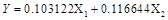where;

Y=investment, X1=Value, X2=Capital

To compare the efficiency of the parameter estimates from the GLS and FGLS models we comparatively study their variances as shown in table 4. Those parameter estimates that have smaller variances are deemed to be more efficient. This is due to their small deviance from the true mean.

Table 4. A Comparison between the Variance and Covariance of GLS and FGLS Estimates.

 Value Capital GLS FGLS GLS FGLS Value 3.0824×10- 5 1.5658×10- 5 -9.0776×10- 5 -3.0587×10- 5 Capital -9.0776×10- 5 -3.0587×10- 5 5.3378×10- 4 1.3317×10- 4

From the results in table 4 we observe that FGLS estimates have smaller variances as compared to the GLS estimates implying that they are more efficient. This can be attributed to the fact that, the GLS estimates are obtained under the assumption of known variance-covariance matrix of the error term which is normally not the case, while for FGLS model the variance-covariance matrix is data driven and therefore, no assumptions were made about its structure.

4.6. Robust Covariance Matrix Estimation Results

4.6.1. Heteroscedasticity Consistent (HC) Estimates

The function vcovHC was used to estimate four types of White’s heteroscedasticity-consistent covariance matrix (known as the sandwich estimator). All the types assume no correlation between errors of different groups while allowing for heteroscedasticity across groups, so that the full covariance matrix of errors is a diagonal matrix.

To determine the most efficient Heteroscedasticity Consistent estimator for all the types, the study compares their standard errors as shown in table 5.The estimator with the smallest standard error is touted to be the most efficient.

Table 5. Standard errors given different types of HC.

 Value Capital HC0 0.007117160* 0.04548306* HC1 0.007149733 0.04569122 HC2 0.007302228 0.04844756 HC3 0.007498911 0.05168857 HC4 0.007884342 0.05898951

Where * indicates the smallest standard error. From the results in table 5, Heteroscedasticity consistent type HC0returned the smallest standard errors for the estimates of both value and capital indicating that it is the most efficient estimator of all the heteroscedasticity consistent types.

4.6.2. Heteroscedasticity and Autocorrelation Consistent (HAC) Estimate

We note that the residuals in our data sets were both heteroscedastic and serially correlated thus leading to an error covariance matrix that is non-diagonal.

We therefore use the function vcovHAC to obtain a heteroscedasticity and autocorrelation consistent (HAC) covariance matrix estimate. The results of this estimate are shown in table 6.

Table 6. Heteroscedasticity and Autocorrelation Consistent (HAC) Estimate.

 Value Capital Value 0.0002133486 -0.0003059557 Capital -0.0003059557 0.0050457394

The square root of the leading diagonal elements of the HAC estimate in table 6 gives the heteroscedasticity and autocorrelation consistent standard errors (HAC-SEs) for the least squares estimates as shown in table 7

Table 7. Standard errors for HAC Estimate.

 Value Capital HAC-SEs 0.014606457 0.071033368

From the results in table 7 we note that HAC-SEs for the parameter estimates are bigger than the biggest HC estimates for the corresponding variables despite considering autocorrelation of the errors. This however, supports the results of the Hausman test on our data sets which implied that endogeneity is not a problem for the random effects estimator.

4.7. Measure of Performance

To find out which model fits our data sets better, the study considered two performance measures; Residual Sum of Squares (RSS) and the Non-parametric R-squared. The results of this assessment are shown in table 8.

Table 8. Performance Measure for the different Models.

 RSS R2 POOLED OLS 1938557 80.58% GLS 2119923 78.29% FGLS 2155038 80.75%

From the results in table 8, 80.58% of the dependent variable (investment) was explained by the regressor variables (value and capital) according to the OLS model fitted under robust covariance estimation (sandwich). This model also returned the smallest residual sum of squares, 1938557, implying a smaller distance between the original observations and the fitted values as compared with the other models. Generalized least squares model was also fitted by specifying the structure of the correlation. 78.29% of the dependent variable was explained by the regressors under this model and the resulting residuals summed to 2119923.

By first estimating the variance covariance matrix of the residuals, feasible generalized least squares model was fitted and 80.75% of the dependent variable was explained by the regressors. However, this model returned the highest residual sum of squares, 2155038, implying a larger deviation between the original observations and the predicted values.

The study therefore concludes that, in terms of the non-parametric R squared, Feasible GLS gives a good fit of our datasets while in terms of residual sum of squares, pooled ordinary least squares performed better than both generalized least squares and feasible generalized least squares.

5. Conclusion and Recommendations

The main aim of this study was to model panel data using the conventional regression based techniques and to compare the efficiency of the estimates returned by the suggested models. We squabble that the use of robust standard estimators instead of ignoring heteroscedasticity and autocorrelation when carrying out inferential tests in OLS regression, guarantees the researcher gratifying comfort in the legitimacy and power of those tests. This is why we argue that robust covariance matrix estimation and generalized least squares methods should be routinely used in panel data analysis to make sure that conclusions drawn on such research works are not compromised by heteroscedasticity.

This study considered the basic panel linear model pooled across all the cross-sections. The scope of this research can therefore, be expanded by considering panel linear models for each cross-section (firm) without pooling them. The detection of heteroscedasticity can also function as an impulsion for future research to realize the process producing heteroscedasticity in this data set.

Nomenclature

FGLS: Feasible Generalized Lest Squares

GLS: Generalized Least Squares

HAC: Heteroscedasticity and Autocorrelation Consistent

HC: Heteroscedasticity Consistent

HCSE: Heteroscedasticity Consistent Standard Errors

OLS: Ordinary Least Squares

References

1. Cribari Neto, F. (2004), "Asymptotic panel inference under heteroscedasticity of unknown form," Computational Statistics and Data Analysis, vol. 45, pp. 215–233., April 1955. (references)
2. Ferrari and Cordeiro (2000), ‘Improved heteroscedasticity consistent covariance matrix estimators’, Biometrika, vol. 87, pp 907-918
3. Grizzle, J. E. and D. M. Allen (1969), ‘Analysis of growth and dose response curves’, Biometrics Vol. 25, pp 357-381
4. Grunfeld, Y (1958), The Determinants of Corporate Investment, PhD thesis, University of Chicago.
5. Hausman, J. (1978), "Specification Tests in Econometrics", Econometrica Vol. 46, pp 1251-1271
6. Kleiber and Zeileis (2010), ‘The Grunfeld data at 50’, German Economic Review.
7. Laird, Nan M. and James H. Ware (1982), ‘Random-effects models for longitudinal data’, Biometrics vol. 38, pp 963-974.
8. Lazarsfeld, Paul F. and Marjorie Fiske (1938), ‘The panel as a new tool for measuring opinion’, Public Opinion Quarterly 2 pp. 596–612
9. Long, J. S and L. Ervin (2000), ‘Using heteroscedasticity-consistent standard errors in the linear regression model’, The American Statistician Vol. 54, pp 217–224.
10. Marschak, J. (1939), "On combining market and budget data in demand studies", Econometrica, Vol. 7, pp 332-335
11. Pesaran, M. (2004), ‘General diagnostic ttest for cross section dependence in panels’, CESinfo Working Papers Series p. 1229
12. Potthoff, R. F. and S. N. (1964) Roy (1964), ‘A generalized multivariate analysis of variance model useful especially for growth curve problems.’, Biometrika Vol. 51, 313–326
13. Rao, C. R. (1965), ‘The theory of least squares when the parameters are stochastic and its application to the analysis of growth curves.’ Biometrika Vol. 52, 447–458.
14. Tobin, J. (1950), "A statistical demand function for food in the U.S.A", Journal of the Royal Statistical Society, Series A, pp 113-141 Vol. 52, 447–458.
15. Wishart, J. (1938), ‘Growth-rate determinations in nutrition studies with the bacon pig, and their analysis.’ Biometrika Vol. 30, 16–28.
16. Wooldridge, J. (2002), ‘Econometric analysis of crosssection and panel data’, MIT press.
17. Zivot, E. and J. Wang (2003), Modeling Financial Time Series with S-plus, Springer-Verlag, New York.

 Contents 1. 2. 3. 3.1. 3.2. 3.3. 4. 4.1. 4.2. 4.3. 4.4. 4.5. 4.6. 4.7. 5.
Article ToolsAbstractPDF(303K)